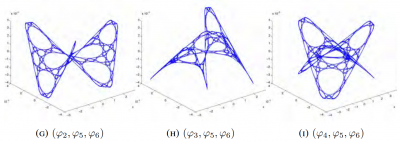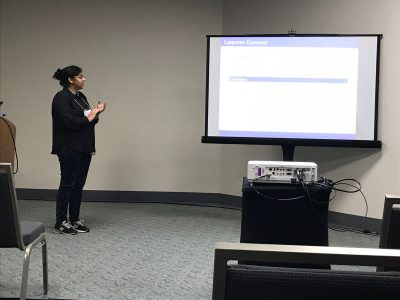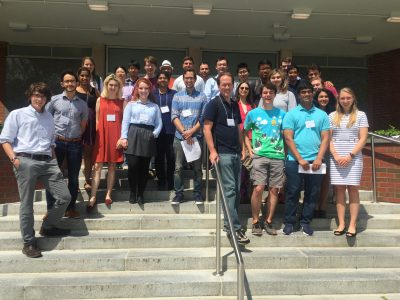# Laplacian eigenmaps 2023

May 18, 2023REU participants:
Bobita Atkins, Massachusetts College of Liberal Arts
Ashka Dalal, Rose-Hulman Institute of Technology
Natalie Dinin, California State University, Chico
Jonathan Kerby-White, Indiana University Bloomington
Tess McGuinness, University of Connecticut
Tonya Patricks, University of Central Florida
Genevieve Romanelli, Tufts University
Yiheng Su, Colby College

Mentors: Bernard Akwei, Rachel Bailey, Luke Rogers, Alexander Teplyaev

Convergence, optimization and stabilization of singular eigenmaps

B.Akwei, B.Atkins, R.Bailey, A.Dalal, N.Dinin, J.Kerby-White, T.McGuinness, T.Patricks, L.Rogers, G.Romanelli, Y.Su, A.Teplyaev

Eigenmaps are important in analysis, geometry and machine learning, especially in nonlinear dimension reduction.

Versions of the Laplacian eigenmaps of Belkin and Niyogi are a widely used nonlinear dimension reduction technique in data analysis.  Data points in a high dimensional space $$\mathbb{R}^N$$ are treated as vertices of a graph, for example by taking edges between points separated by distance at most a threshold $$\epsilon$$ or by joining each vertex to its $$k$$ nearest neighbors.  A small number $$D$$ of eigenfunctions of the graph Laplacian are then taken as coordinates for the data, defining an eigenmap to $$\mathbb{R}^D$$. This method was motivated by an intuitive argument suggesting that if the original data consisted of $$n$$ sufficiently well-distributed points on a nice manifold $$M$$ then the eigenmap would preserve geometric features of $$M$$.

Several authors have developed rigorous results on the geometric properties of eigenmaps, using a number of different assumptions on the manner in which the points are distributed, as well as hypotheses involving, for example, the smoothness of the manifold and bounds on its curvature.  Typically, they use the idea that under smoothness and curvature assumptions one can approximate the Laplace-Beltrami operator of $$M$$ by an operator giving the difference of the function value and its average over balls of a sufficiently small size $$\epsilon$$, and that this difference operator can be approximated by graph Laplacian operators provided that the $$n$$ points are sufficiently well distributed.

In the present work we consider several model situations where eigen-coordinates can be computed analytically as well as numerically, including the intervals with uniform and weighted measures, square, torus, sphere, and the Sierpinski gasket.  On these examples we investigate the connections between eigenmaps and orthogonal polynomials, how to determine the optimal value of $$\epsilon$$ for a given $$n$$ and prescribed point distribution, and the dependence and stability of the method when the choice of Laplacian is varied.  These examples are intended to serve as model cases for later research on the corresponding problems for eigenmaps on weighted Riemannian manifolds, possibly with boundary, and on some metric measure spaces, including fractals.

Approximation of the eigenmaps of a Laplace operator depends crucially on the scaling parameter $$\epsilon$$. If $$\epsilon$$ is too small or too large, then the approximation is inaccurate or completely breaks down. However, an analytic expression for the optimal $$\epsilon$$ is out of reach. In our work, we use some explicitly solvable models and Monte Carlo simulations to find the approximately optimal value of $$\epsilon$$ that gives, on average, the most accurate approximation of the eigenmaps.

Our study is primarily inspired by the work of Belkin and Niyogi   “Towards a theoretical foundation for Laplacian-based manifold methods.”

Results are presented at the 2023 Young Mathematicians Conference (YMC) at the Ohio State University, a premier annual conference for undergraduate research in mathematics, and at the 2024 Joint Mathematics Meetings (JMM) in San Francisco, the largest mathematics gathering in the world.

# Fractional Gaussian fields on surfaces and graphs

March 22, 2023

Group Members: Tyler Campos, Andrew Gannon, Benjamin Hanzsek-Brill, Connor Marrs, Alexander Neuschotz, Trent Rabe and Ethan Winters.

Overview: We study and simulate on computers the fractional Gaussian fields and their discretizations on surfaces like the two-dimensional sphere or two-dimensional torus. The study of the maxima of those processes will be done and conjectures formulated concerning limit laws. Particular attention will be paid to log-correlated fields (the so-called Gaussian free field).

# Fair pricing and hedging under small perturbations of the numéraire on a finite probability space

July 6, 2022

### William Busching, Delphine Hintz, Oleksii Mostovyi, Alexey Pozdnyakov

• Fair Pricing and Hedging Under Small Perturbations of the Numéraire on a Finite Probability Space

Involve (2022), Vol. 15(4), pp. 649-668. [published version] [arXiv]

# The Information Premium on a Finite Probability Space

July 9, 2020

### Jake Koerner, Joo Seung Lee, Oleksii Mostovyi

preprint

accepted in the Missouri Journal of Mathematical Sciences (2023)

# Hedging by Sequential Regression in Generalized Discrete Models and the Follmer-Schweizer decomposition

August 3, 2019## Overview

In practice, financial models are not exact — as in any field, modeling based on real data introduces some degree of error. However, we must consider the effect error has on the calculations and assumptions we make on the model.  In complete markets, optimal hedging strategies can be found for derivative securities; for example, the recursive hedging formula introduced in Steven Shreve’s “Stochastic Calculus for Finance I” gives an exact expression in the binomial asset model, and as a result the unique arbitrage-free price can be computed at any time for any derivative security.

In incomplete markets this cannot be accomplished; one possibility for computing optimal hedging strategies is the method of sequential regression.  We considered this in discrete-time; in the (complete) binomial model we showed that the strategy of sequential regression introduced by Follmer and Schweizer  is equivalent to Shreve’s recursive hedging formula, and in the (incomplete) trinomial model we both explicitly computed the optimal hedging strategy predicted by the Follmer-Schweizer decomposition and we showed that the strategy is stable under small perturbations.

# Math UConn REU at JMM 2018

January 6, 2018

Two of our REU (2017 Stochastics) participants, Raji Majumdar and Anthony Sisti, will be presenting posters Applications of Multiplicative LLN and CLT for Random Matrices and Black Scholes using the Central Limit Theorem on Friday, January 12 at the MAA Student Poster Session, and both of them will be giving talks on Saturday, January 13 at the AMS Contributed Paper Session on Research in Applied Mathematics by Undergraduate and Post-Baccalaureate Students.

Their travel to the 2018 JMM has been made possible with the support of the MAA and UConn’s OUR travel grants.# 5th Northeast Mathematics Undergraduate Research Mini-Symposium

August 17, 2017

### University of Connecticut, August 3rd, 2017

#### Participating Schools: Amherst, Smith, UConn and UMass

5th Mini-Symposium full program (2017)# Multiplicative LLN and CLT and their Applications

July 25, 2017

## Overview

We study the Law of Large Numbers (LLN) and and Central Limit Theorems (CLT) for products of random matrices. The limit of the multiplicative LLN is called the Lyapunov exponent. We perturb the random matrices with a parameter and we look to find the dependence of the the Lyapunov exponent on this parameter. We also study the variance related to the multiplicative CLT. We prove and conjecture asymptotics of various parameter dependent plots.

## Presentations:

Raji Majumdar and Anthony Sisti, will present posters Applications of Multiplicative LLN and CLT for Random Matrices and Black Scholes using the Central Limit Theorem on Friday, January 12 at the MAA Student Poster Session, and give talks on Saturday, January 13 at the AMS Contributed Paper Session on Research in Applied Mathematics by Undergraduate and Post-Baccalaureate Students.